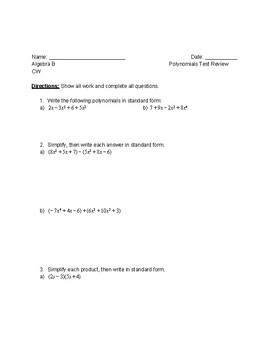# Polynomials and Factoring Review and TestSubject
Resource Type
File Type

PDF

(146 KB|9 pages)
Standards
• Product Description
• Standards
This is a note packet, classwork, and a test for Polynomials and Factoring. This can be used for various Algebra classes. Goes through standard form of polynomials, adding and subtracting polynomials, and factoring.
Know and apply the Remainder Theorem: For a polynomial 𝘱(𝘹) and a number 𝘢, the remainder on division by 𝘹 – 𝘢 is 𝘱(𝘢), so 𝘱(𝘢) = 0 if and only if (𝘹 – 𝘢) is a factor of 𝘱(𝘹).
Understand that polynomials form a system analogous to the integers, namely, they are closed under the operations of addition, subtraction, and multiplication; add, subtract, and multiply polynomials.
Total Pages
9 pages
N/A
Teaching Duration
N/A
Report this Resource to TpT
Reported resources will be reviewed by our team. Report this resource to let us know if this resource violates TpT’s content guidelines.